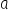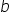Mathematics
Easy

Question

# Which properties  can be used to add 18.25 + 7.99 + 6.75

## Commutative & AssociativeAssociative & CompensationAssociative @ commutativeCompensationHint:

## The correct answer is: Commutative & Associative

### Now as we know that here we have to find the step by breaking one number. The fact that there are no steps to memorise is one of the best things about mental math. The "friendly number" addition approach facilitates working with large numbers. This is due to the fact that we are, in essence, decomposing the problem into more manageable components.Here we will use some properties which are: Commutative Property of Addition: if aand bare real numbers, then a+b=b+a Associative Property of Addition: if a,b, and c are real numbers, then (a+b)+c=a+(b+c) Now we have given 18.25 + 7.99 + 6.75, let's solve this.18.25 + 7.99 + 6.7518.25 + (6.75 + 7.99) ( using commutative property)(18.25 + 6.75) + 7.99 ( using the associative property)25 + 7.99 = 32.99So, the sum is 32.99

Here the concept of Break Apart Numbers is used where a number is divided in two parts to make addition easy. The concept of friendly numbers is also used so that it becomes easy for addition or subtraction purpose. Some properties like associative and commutative properties are also used to solve easily. So, the sum is 32.99

### Related Questions to study#### With Turito Foundation.#### Get an Expert Advice From Turito.